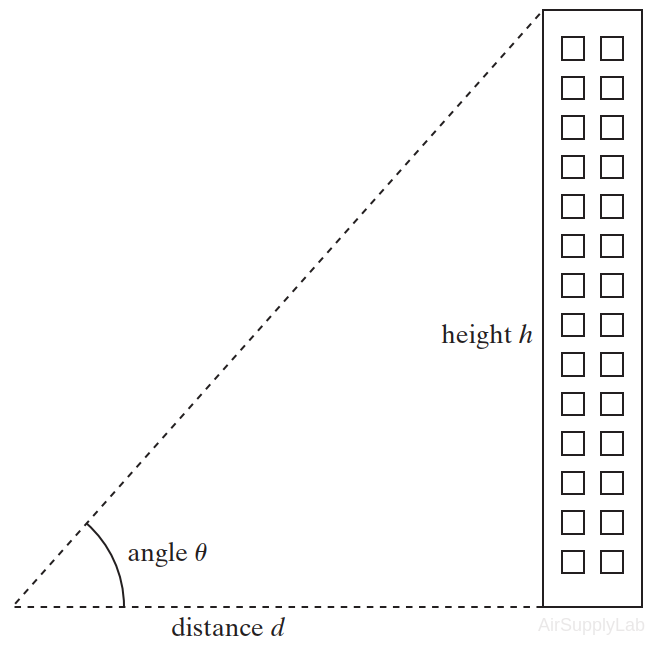# Lesson 02: Built-In MATLAB Functions

## Objectives

• To use a variety of common mathematical functions.
• To understand and use trigonometric function in MATLAB.
• Recognize and be able to use the special values and functions built into MATLAB.

## Background

MATLAB includes many built-in functions for math operations. Here are a number of the most important ones. A big advantage of MATLAB is that function arguments can generally be either scalars or arrays.

### Elementary Math Functions

Elementary math functions include logarithms, exponentials, absolute value, rounding functions, and functions used in discrete mathematics.

## Questions

Use MATLAB functions or commands to solve the questions.

1. Populations tend to expand exponentially, that is,where
P = current population
P0 = original population
r = continuous growth rate expressed as a fraction
t = time.
If you originally have 100 rabbits that breed at a continuous growth rate of 90% ( r = 0.92) per year, find how many rabbits you will have at the end of 10 years.
2. You can use trigonometry to find the height of a building as shown in the following Figure.Suppose you measure the angle between the line of sight and the horizontal line connecting the measuring point and the building. You can calculate the height of the building with the following formulas:Assume that the distance to the building along the ground is 120 m and the angle measured along the line of sight is 30° ± 3°. Find the maximum and minimum heights the building can be.